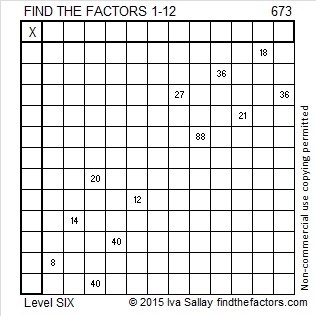# 673 and Level 6

673 = 23² + 12².

673 is the hypotenuse of the primitive Pythagorean triple 385-552-673 which was calculated using 23² – 12², 2(23)(12), 23² + 12².Print the puzzles or type the solution on this excel file: 12 Factors 2015-11-02

—————————————————————————————————

• 673 is a prime number.
• Prime factorization: 673 is prime.
• The exponent of prime number 673 is 1. Adding 1 to that exponent we get (1 + 1) = 2. Therefore 673 has exactly 2 factors.
• Factors of 673: 1, 673
• Factor pairs: 673 = 1 x 673
• 673 has no square factors that allow its square root to be simplified. √673 ≈ 25.94224.How do we know that 673 is a prime number? If 673 were not a prime number, then it would be divisible by at least one prime number less than or equal to √673 ≈ 25.9. Since 673 cannot be divided evenly by 2, 3, 5, 7, 11, 13, 17, 19, or 23, we know that 673 is a prime number.

Here’s another way we know that 673 is a prime number: Since 673÷4 has a remainder of one, and 23² + 12² = 673, and 23 and 12 have no common prime factors, 673 will be prime unless it is divisible by a primitive Pythagorean hypotenuse less than or equal to √673 ≈ 25.9. Since 673 is not divisible by 5, 13, or 17, we know that 673 is a prime number.Don’t get discouraged if you make a mistake. Just fix it and move on. That’s what Ricardo did: